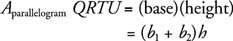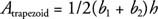## Trapezoids

Probably the trapezoid is one of the most popular quadrilaterals when it comes to bridge construction. Numerous railroad trestles and wooden bridges of the nineteenth and early twentieth centuries were trapezoidal in shape.

In Figure 1, trapezoid QRSV is labeled so that b1 and b2 are the bases (h is the height to these bases) and a and c are the legs. The perimeter is simply the sum of these lengths.Figure 1 A trapezoid and the associated parallelogram.

Referring to Figure , an identical, but upside‐down trapezoid is drawn adjacent to trapezoid QRSV, trapezoid TUVS. It can now be shown that the figure QRTU is a parallelogram, and its area can now be found.Because trapezoid QRSV is exactly half of this parallelogram, the following formula gives the area of a trapezoid.Example 1: Find the perimeter and area of Figure 2.Figure 2 Finding the perimeter and area of a trapezoid.

The figure is a trapezoid.